# Meat

This item is of food class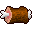You see a piece of meat.
Attributes
AMOUNT
60
FREQUENCY
20
REGEN
5
Eat|Use
Dropped by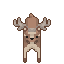deer
Drops between 1 and 3.
Probability: 60%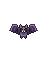bat
Drops between 1 and 2.
Probability: 50%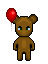babybear
Drops between 1 and 2.
Probability: 50%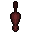beaver
Drops between 1 and 2.
Probability: 80%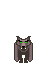big bad wolf
Drops between 1 and 4.
Probability: 60%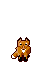fox
Drops between 1 and 4.
Probability: 60%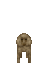monkey
Drops between 1 and 4.
Probability: 60%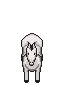white horse
Drops between 1 and 2.
Probability: 80%wolf
Drops between 1 and 4.
Probability: 60%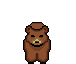bear
Drops between 3 and 7.
Probability: 50%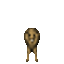lion
Drops between 2 and 6.
Probability: 60%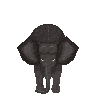elephant
Drops between 1 and 10.
Probability: 100%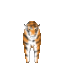tiger
Drops between 1 and 6.
Probability: 60%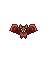killer bat
Drops between 1 and 2.
Probability: 80%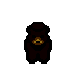black bear
Drops between 3 and 12.
Probability: 60%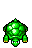turtle
Drops between 1 and 5.
Probability: 20%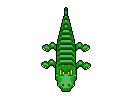crocodile
Drops between 3 and 7.
Probability: 80%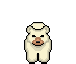polar bear
Drops between 5 and 15.
Probability: 60%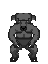sheepman
Drops between 1 and 5.
Probability: 10%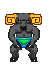elite sheepman
Drops between 2 and 6.
Probability: 22.5%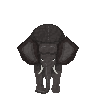bull elephant
Drops between 1 and 10.
Probability: 100%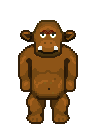cave troll
Drops between 1 and 5.
Probability: 10.5%
Quests
© 1999-2021 Arianne Project
Server time: 21:15